## General Chemistry

In a precipitation reaction, an insoluble product is formed which is called a precipitate. Precipitation reactions usually involve ionic compounds. For example, when an aqueous solution of sodium chromate (Na2CrO4) is added to an aqueous solution of silver nitrate (AgNO3), a dark orange precipitate of silver chromate (Ag2CrO4) is formed:

Na2CrO4(aq) + AgNO3(aq) → Ag2CrO4(s) + NaNO3(aq)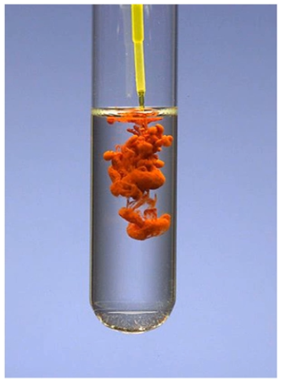# Predicting the Products of a Reaction

If we compare the formulas of the reactants and products in the previous reaction, we can see that an exchange of ions had occurred; the cation of one salt combined with the anion of the other salt and vice versa. These types of reactions are called metathesis or double-displacement reactions.

To be able to predict the products of a double displacement reaction, you need to know the formulas and the charges of the ions in the starting materials and use the solubility rules to identify the precipitate in the reaction.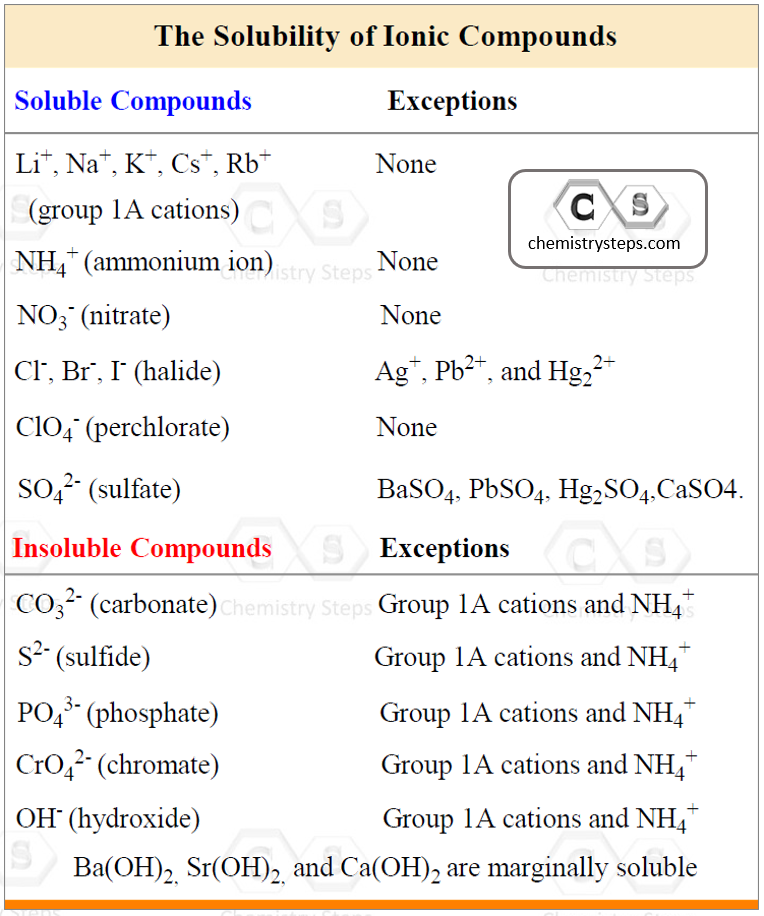Let’s use the table of the most common polyatomic ions, and determine the products of the reaction between AgNOand CaCl2: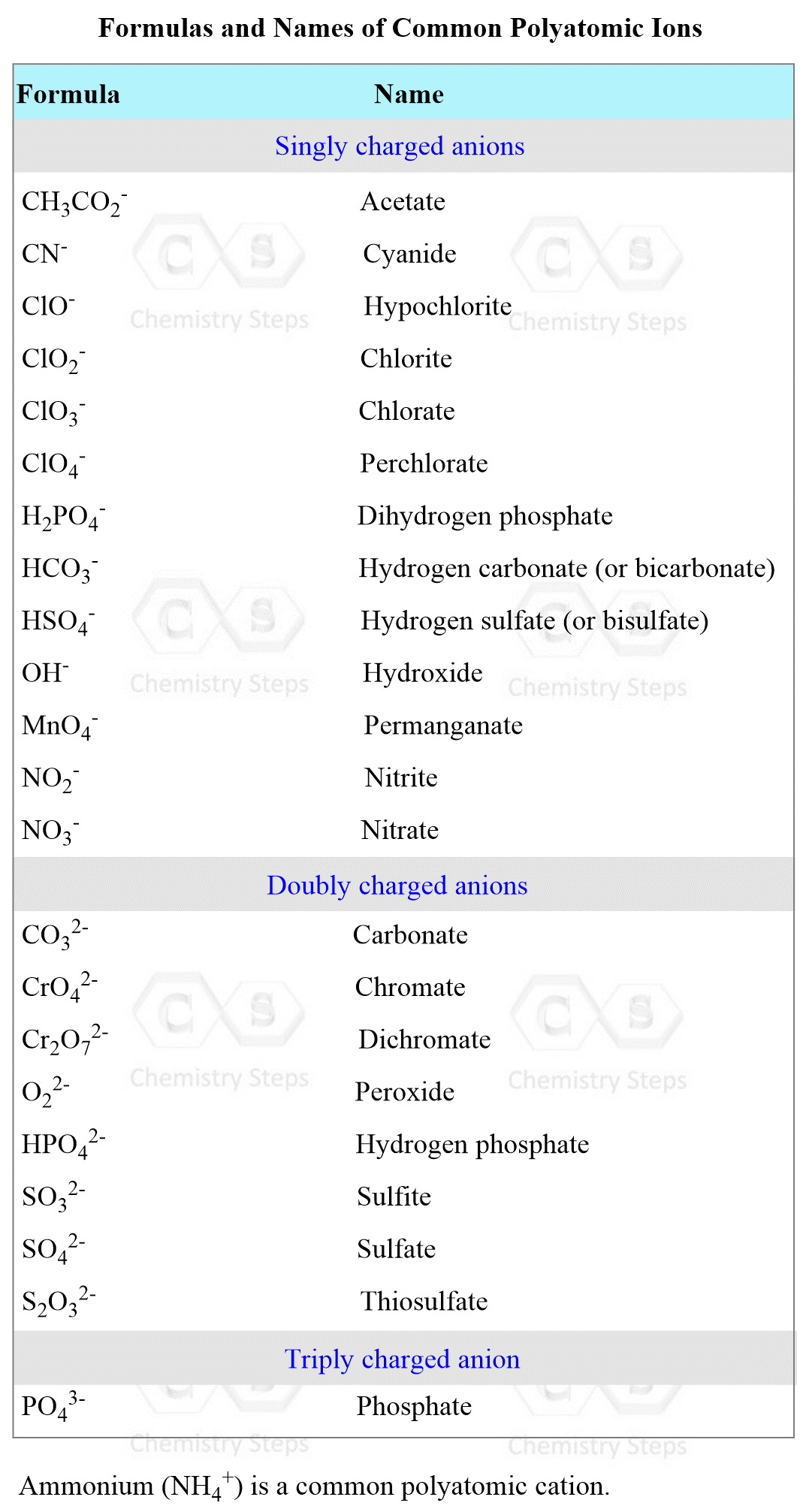Complete and balance the following equation between solver nitrate and calcium chloride:

AgNO3(aq) + CaCl2(aq) → ?

1) Exchange the cations and anions. Only carry the subscripts that are part of the formula. I.e., we don’t carry the subscript of Cl, but we do for the nitrate since the formulas are Cl and NO3: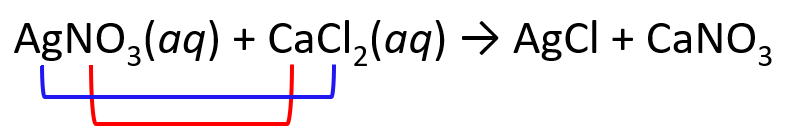2) Place the ionic charges on top of elements and adjust the subscripts: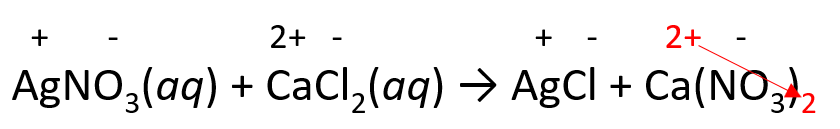3) Balance the equation with coefficients and remove the charges:

2AgNO3(aq) + CaCl2(aq) → 2AgCl + Ca(NO3)2

4) Label the soluble compounds as (aq). Determine the precipitate (in this case AgCl) and label it as (s) – solid.

2AgNO3(aq) + CaCl2(aq) → 2AgCl(s) + Ca(NO3)2(aq)

AgCl is a solid (precipitate) because the salts of halides are soluble except when combined with Ag+, Pb2+, and Hg22+.

Being able to predict the products of a reaction is very important partly because it is required for solving problems on determining the amount of the product based on the stoichiometry of the reaction.

In the next post, we will talk about the stoichiometry of reactions in aqueous solutions and examples of precipitation and acid-base reactions.

Check Also

#### Practice

1.

Complete and balance each of the following molecular equations in aqueous solution:

a) AgNO3(aq) + CaCl2(aq) →

b) HNO3(aq) + Na2CO3(aq) →

c) K2CO3(s) + HClO3(aq) →

d) Al(OH)3(s) + HBr(aq) →

e) H3PO4(aq) + Fe(OH)3(s) →

f) BaCl2(aq) + K2SO4(aq) →

a)

a) 2AgNO3(aq) + CaCl2(aq) → 2AgCl(s) + Ca(NO3)2(aq)

b)

b) 2HNO3(aq) + Na2CO3(aq) → 2NaNO3(aq) + CO2(g)+ H2O(l)

c)

c) K2CO3(s) + HClO3(aq) → 2KClO3(aq) + CO2(g)+ H2O(l)

d)

d) Al(OH)3(s) + 3HBr(aq) → AlBr3(aq) + 3H2O(l)

e)

e) H3PO4(aq) + Fe(OH)3(s) → FePO4(s) + 3H2O(l)

f)

f) BaCl2(aq) + K2SO4(aq) → BaSO4(s) + 2KCl(aq)

Solution

We predict the products by combining the cation from each reactant with the anion from the other. To obtain correct formulas, make sure you know the charge of the cation and the formula together with the charge of the anion. Adjust the subscript based on the charges and remember, the overall charge of the compound should balance to zero.

So, let’s make a list of steps for predicting the products of ionic reactions.

1) Identify the ions and write their charge on top

1a) Combine the cations and anions

2) Exchange the charges as subscripts

2a) Balance the equation and omit the charges

_____________________________________________________________________________

a) AgNO3(aq) + PbCl2(aq) →

1) Combine the cations and anions:2) Balance the equation and omit the charges:

2AgNO3(aq) + CaCl2(aq) → 2AgCl(s) + Ca(NO3)2(aq)

b) HNO3(aq) + Na2CO3(aq) →

1) Combine the cations and anions:2) Balance the equation and omit the charges:

Remember that carbonic acid is unstable and decomposes immediately to CO2(g)+ H2O(l):

H2CO3(aq) → CO2(g)+ H2O(l)

So, in the final/corrected version of the equation, we write CO2 and H2O and not H2COwhich we use here to demonstrate how the combination of ions occurs.

2HNO3(aq) + Na2CO3(aq) → 2NaNO3(aq) + CO2(g)+ H2O(l)

c) K2CO3(s) + HClO3(aq) →

1) Combine the cations and anions:2) Balance the equation and omit the charges:

Remember that carbonic acid is unstable and decomposes immediately to CO2(g)+ H2O(l):

H2CO3(aq) → CO2(g)+ H2O(l)

So, in the final/corrected version of the equation, we write CO2 and H2O and not H2COwhich we use here to demonstrate how the combination of ions occurs.

K2CO3(s) + HClO3(aq) → 2KClO3(aq) + CO2(g)+ H2O(l)

d) Al(OH)3(s) + HBr(aq) →

1) Combine the cations and anions:2) Balance the equation and omit the charges:

Al(OH)3(s) + 3HBr(aq) → AlBr3(aq) + 3H2O(l)

Notice that HOH is a result of combining H+ and OH, and it is the same as H2O.

e) H3PO4(aq) + Fe(OH)3(s) →

1) Combine the cations and anions:2) Balance the equation and omit the charges:

H3PO4(aq) + Fe(OH)3(s) → FePO4(s) + 3H2O(l)

f) BaCl2(aq) + K2SO4(aq) →

1) Combine the cations and anions:2) Balance the equation and omit the charges:

BaCl2(aq) + K2SO4(aq) → BaSO4(s) + 2KCl(aq)

### 1 thought on “Precipitation Reactions”

1.Thanks for the great work you do